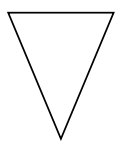# Acute, Obtuse, and Right Triangles Online Quiz

Following quiz provides Multiple Choice Questions (MCQs) related to Acute, Obtuse, and Right Triangles. You will have to read all the given answers and click over the correct answer. If you are not sure about the answer then you can check the answer using Show Answer button. You can use Next Quiz button to check new set of questions in the quiz.Q 1 - Identify the given triangle as acute, obtuse or right triangle.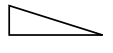### Explanation

Step 1:

One of the angles of the given triangle is a right angle.

Step 2:

So the given triangle is a right triangle.

Q 2 - Identify the given triangle as acute, obtuse or right triangle.### Explanation

Step 1:

All angles of the given triangle are acute.

Step 2:

So the given triangle is an acute triangle.

Q 3 - Identify the given triangle as acute, obtuse or right triangle.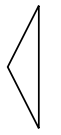### Explanation

Step 1:

One of the angles of the given triangle is an obtuse angle.

Step 2:

So the given triangle is an obtuse triangle.

Q 4 - Identify the given triangle as acute, obtuse or right triangle.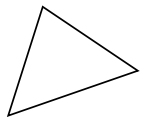### Explanation

Step 1:

All angles of the given triangle are acute.

Step 2:

So the given triangle is an acute triangle.

Q 5 - Identify the given triangle as acute, obtuse or right triangle.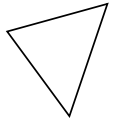### Explanation

Step 1:

All angles of the given triangle are acute.

Step 2:

So the given triangle is an acute triangle.

Q 6 - Identify the given triangle as acute, obtuse or right triangle.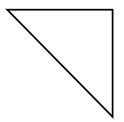### Explanation

Step 1:

One of the angles of the given triangle is a right angle.

Step 2:

So the given triangle is a right triangle.

Q 7 - Identify the given triangle as acute, obtuse or right triangle.### Explanation

Step 1:

One of the angles of the given triangle is a right angle.

Step 2:

So the given triangle is a right triangle.

Q 8 - Identify the given triangle as acute, obtuse or right triangle.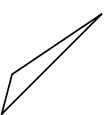### Explanation

Step 1:

One of the angles of the given triangle is an obtuse angle.

Step 2:

So the given triangle is an obtuse triangle.

Q 9 - Identify the given triangle as acute, obtuse or right triangle.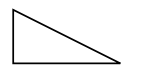### Explanation

Step 1:

One of the angles of the given triangle is a right angle.

Step 2:

So the given triangle is a right triangle.

Q 10 - Identify the given triangle as acute, obtuse or right triangle.